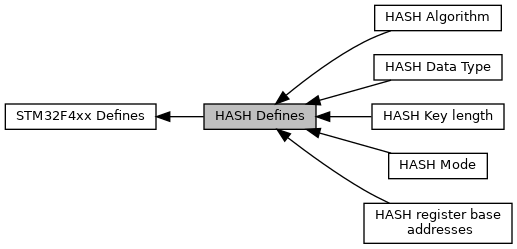libopencm3 A free/libre/open-source firmware library for various ARM Cortex-M3 microcontrollers.
HASH Defines

Defined Constants and Types for the STM32F4xx HASH Controller. More...

Collaboration diagram for HASH Defines:HASH Data Type

HASH Mode

HASH Algorithm

HASH Key length

## Macros

#define HASH_CR   MMIO32(HASH + 0x00)

#define HASH_DIN   MMIO32(HASH + 0x04)

#define HASH_STR   MMIO32(HASH + 0x08)

#define HASH_HR   (&MMIO32(HASH + 0x0C)) /* x5 */

#define HASH_IMR   MMIO32(HASH + 0x20)

#define HASH_SR   MMIO32(HASH + 0x28)

#define HASH_CSR   (&MMIO32(HASH + 0xF8)) /* x51 */

#define HASH_CR_INIT   (1 << 2)

#define HASH_CR_DMAE   (1 << 3)

#define HASH_CR_DATATYPE   (3 << 4)

#define HASH_CR_MODE   (1 << 6)

#define HASH_CR_ALGO   (1 << 7)

#define HASH_CR_NBW   (15 << 8)

#define HASH_CR_DINNE   (1 << 12)

#define HASH_CR_LKEY   (1 << 16)

#define HASH_STR_NBW   (31 << 0)

#define HASH_STR_DCAL   (1 << 8)

#define HASH_IMR_DINIE   (1 << 0)

#define HASH_IMR_DCIE   (1 << 1)

#define HASH_SR_DINIS   (1 << 0)

#define HASH_SR_DCIS   (1 << 1)

#define HASH_SR_DMAS   (1 << 2)

#define HASH_SR_BUSY   (1 << 3)

## Functions

void hash_set_mode (uint8_t mode)
HASH Set Mode. More...

void hash_set_algorithm (uint8_t algorithm)
HASH Set Algorithm. More...

void hash_set_data_type (uint8_t datatype)
HASH Set Data Type. More...

void hash_set_key_length (uint8_t keylength)
HASH Set Key Length. More...

void hash_set_last_word_valid_bits (uint8_t validbits)
HASH Set Last Word Valid Bits. More...

void hash_init (void)
HASH Init. More...

void hash_digest (void)
HASH Digest. More...

void hash_get_result (uint32_t *data)
HASH Get Hash Result. More...

## Detailed Description

Defined Constants and Types for the STM32F4xx HASH Controller.

Version
1.0.0
Date
31 May 2013

## ◆ HASH_CR

 #define HASH_CR   MMIO32(HASH + 0x00)

Definition at line 48 of file hash_common_f24.h.

## ◆ HASH_CR_ALGO

 #define HASH_CR_ALGO   (1 << 7)

Definition at line 109 of file hash_common_f24.h.

## ◆ HASH_CR_DATATYPE

 #define HASH_CR_DATATYPE   (3 << 4)

Definition at line 87 of file hash_common_f24.h.

## ◆ HASH_CR_DINNE

 #define HASH_CR_DINNE   (1 << 12)

Definition at line 115 of file hash_common_f24.h.

## ◆ HASH_CR_DMAE

 #define HASH_CR_DMAE   (1 << 3)

Definition at line 74 of file hash_common_f24.h.

## ◆ HASH_CR_INIT

 #define HASH_CR_INIT   (1 << 2)

Definition at line 71 of file hash_common_f24.h.

## ◆ HASH_CR_LKEY

 #define HASH_CR_LKEY   (1 << 16)

Definition at line 126 of file hash_common_f24.h.

## ◆ HASH_CR_MODE

 #define HASH_CR_MODE   (1 << 6)

Definition at line 98 of file hash_common_f24.h.

## ◆ HASH_CR_NBW

 #define HASH_CR_NBW   (15 << 8)

Definition at line 112 of file hash_common_f24.h.

## ◆ HASH_CSR

 #define HASH_CSR   (&MMIO32(HASH + 0xF8)) /* x51 */

Definition at line 66 of file hash_common_f24.h.

## ◆ HASH_DIN

 #define HASH_DIN   MMIO32(HASH + 0x04)

Definition at line 51 of file hash_common_f24.h.

## ◆ HASH_HR

 #define HASH_HR   (&MMIO32(HASH + 0x0C)) /* x5 */

Definition at line 57 of file hash_common_f24.h.

## ◆ HASH_IMR

 #define HASH_IMR   MMIO32(HASH + 0x20)

Definition at line 60 of file hash_common_f24.h.

## ◆ HASH_IMR_DCIE

 #define HASH_IMR_DCIE   (1 << 1)

Definition at line 143 of file hash_common_f24.h.

## ◆ HASH_IMR_DINIE

 #define HASH_IMR_DINIE   (1 << 0)

Definition at line 140 of file hash_common_f24.h.

## ◆ HASH_SR

 #define HASH_SR   MMIO32(HASH + 0x28)

Definition at line 63 of file hash_common_f24.h.

## ◆ HASH_SR_BUSY

 #define HASH_SR_BUSY   (1 << 3)

Definition at line 157 of file hash_common_f24.h.

## ◆ HASH_SR_DCIS

 #define HASH_SR_DCIS   (1 << 1)

Definition at line 151 of file hash_common_f24.h.

## ◆ HASH_SR_DINIS

 #define HASH_SR_DINIS   (1 << 0)

Definition at line 148 of file hash_common_f24.h.

## ◆ HASH_SR_DMAS

 #define HASH_SR_DMAS   (1 << 2)

Definition at line 154 of file hash_common_f24.h.

## ◆ HASH_STR

 #define HASH_STR   MMIO32(HASH + 0x08)

Definition at line 54 of file hash_common_f24.h.

## ◆ HASH_STR_DCAL

 #define HASH_STR_DCAL   (1 << 8)

Definition at line 135 of file hash_common_f24.h.

## ◆ HASH_STR_NBW

 #define HASH_STR_NBW   (31 << 0)

Definition at line 132 of file hash_common_f24.h.

## Function Documentation

 void hash_add_data ( uint32_t data )

Puts data into the HASH processor's queue.

Parameters
 [in] data unsigned int32. Hash input data.

Definition at line 127 of file hash_common_f24.c.

References HASH_DIN.

## ◆ hash_digest()

 void hash_digest ( void )

HASH Digest.

Starts the processing of the last data block.

Definition at line 139 of file hash_common_f24.c.

References HASH_STR, and HASH_STR_DCAL.

## ◆ hash_get_result()

 void hash_get_result ( uint32_t * data )

HASH Get Hash Result.

Makes a copy of the resulting hash.

Parameters
 [out] data unsigned int32. Hash 4\5 words long depending on the algorithm.

Definition at line 152 of file hash_common_f24.c.

References HASH_ALGO_SHA1, HASH_CR, HASH_CR_ALGO, and HASH_HR.

## ◆ hash_init()

 void hash_init ( void )

HASH Init.

Initializes the HASH processor.

Definition at line 114 of file hash_common_f24.c.

References HASH_CR, and HASH_CR_INIT.

## ◆ hash_set_algorithm()

 void hash_set_algorithm ( uint8_t algorithm )

HASH Set Algorithm.

Sets up the specified algorithm - either MD5 or SHA1.

Parameters
 [in] algorithm unsigned int8. Hash algorithm: HASH Algorithm

Definition at line 59 of file hash_common_f24.c.

References HASH_CR, and HASH_CR_ALGO.

## ◆ hash_set_data_type()

 void hash_set_data_type ( uint8_t datatype )

HASH Set Data Type.

Sets up the specified data type: 32Bit, 16Bit, 8Bit, Bitstring.

Parameters
 [in] datatype unsigned int8. Hash data type: HASH Data Type

Definition at line 73 of file hash_common_f24.c.

References HASH_CR, and HASH_CR_DATATYPE.

## ◆ hash_set_key_length()

 void hash_set_key_length ( uint8_t keylength )

HASH Set Key Length.

Sets up the specified key length: Long, Short.

Parameters
 [in] keylength unsigned int8. Hash data type: HASH Key length

Definition at line 87 of file hash_common_f24.c.

References HASH_CR, and HASH_CR_LKEY.

## ◆ hash_set_last_word_valid_bits()

 void hash_set_last_word_valid_bits ( uint8_t validbits )

HASH Set Last Word Valid Bits.

Specifies the number of valid bits in the last word.

Parameters
 [in] validbits unsigned int8. Number of valid bits.

Definition at line 101 of file hash_common_f24.c.

References HASH_STR, and HASH_STR_NBW.

## ◆ hash_set_mode()

 void hash_set_mode ( uint8_t mode )

HASH Set Mode.

Sets up the specified mode - either HASH or HMAC.

Parameters
 [in] mode unsigned int8. Hash processor mode: HASH Mode

Definition at line 45 of file hash_common_f24.c.

References HASH_CR, and HASH_CR_MODE.